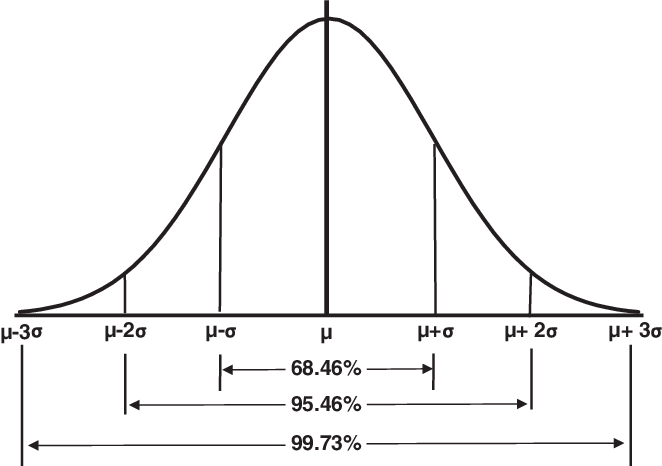# Anybody help!

The average amount of snow that falls during January in Frostbite Falls is normally distributed with a mean of 35 inches and a standard deviation of 5 inches. The probability that the snowfall amount in January of next year will be between 40 inches and 26.75 inches is closest to:

A)

79%.B)

68%.C)

87%.Explanation

To calculate this answer, we will use the properties of the standard normal distribution. First, we will calculate the Z-value for the upper and lower points and then we will determine the approximate probability covering that range. Note: This question is an example of why it is important to memorize the general properties of the normal distribution.

Z = (observation – population mean) / standard deviation

• Z26.75 = (26.75 – 35) / 5 = -1.65. (1.65 standard deviations to the left of the mean)
• Z40 = (40 – 35) / 5 = 1.0 (1 standard deviation to the right of the mean)

Using the general approximations of the normal distribution:

• 68% of the observations fall within ± one standard deviation of the mean. So, 34% of the area falls between 0 and +1 standard deviation from the mean.
• 90% of the observations fall within ± 1.65 standard deviations of the mean. So, 45% of the area falls between 0 and +1.65 standard deviations from the mean.

Here , we have 34% to the right of the mean and 45% to the left of the mean, for a total of 79%.

(Study Session 3, Module 9.2, LOS 9.l)

Hi I don’t understand how to get the 68%, can anybody help, much thanks.Let N(x) be the Cumulative distribution of the (Standard) Normal Probability Function, where x is the number of standard deviations away from the mean. Then:
the probability that the result is within 1 standard deviation is
N(1) - N(-1) = .84134 - .15866 = .68268, or 68.268%

the probability that the result is within 2 standard deviation is
N(2) - N(-2) = .97725 - .02275 = .95450, or 95.45%

the probability that the result is within 3 standard deviation is
N(3) - N(-3) = .99865 - .00135 = .9973, or 99.73%

you can use any statistical program to calculate N(x), or use a Normal Probability Table.

Excel has built-in normal distribution functions:

• NORM.DIST
• NORM.INV
• NORM.S.DIST
• NORM.S.INV

and others.

You can also use tables. If you need a table on the exam, an excerpt will be provided for you.

1 Like

by the way, for a more accurate solution, you would compute
N(1) - N(-1.65) = .84134 - .04947 = .79187 or 79.187%

The EPS of stocks in a large market was found to have a mean of \$12.50 and standard deviation of \$32. The distribution can approximated to a normal distribution.

Sort the value of these from highest to lowest.

• F[44.5]-F[12.5]

• F[-19.5]

• F[76.5]-F[44.5]

Incorrect

F[44.5]-F[12.5] = F(1)-F(0) = 0.8413-0.5 = 0.3413
F[-19.5] = F(-1) = = 0.1587
F[76.5]-F[44.5] = F(2)-F(1) = 0.9772-0.8413 = 0.1359

Hi can anybody explain how do I get 44.5 to F(1), F(12.5) to 0.5, F(19.5) to F(-1)
F(76.5) to F(2).

F(x) = Pr(EPS <=x) = Pr [z<=(x - 12.5)/32] where z is the standard normal distribution.

I added this sentence because system.You are given that distribution is Normal and you know that the mean is 12.50 and the deviation is 32. When dealing with Normal distribution, the first step is to convert it to Standard Normal using the formula (tip: memorize it):

so, for example, when x = 44.5,
z = (44.5 - 12.5)/32 = 1
and using the Cumulative Normal Probability Calculator (or a Normal Probability Table), you’ll find N(1) = .84134

similarly, when x = 12.5,
z = (12.5 - 12.5)/32 = 0
and N(0) = .5 (exactly)